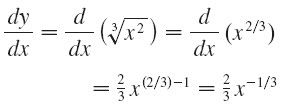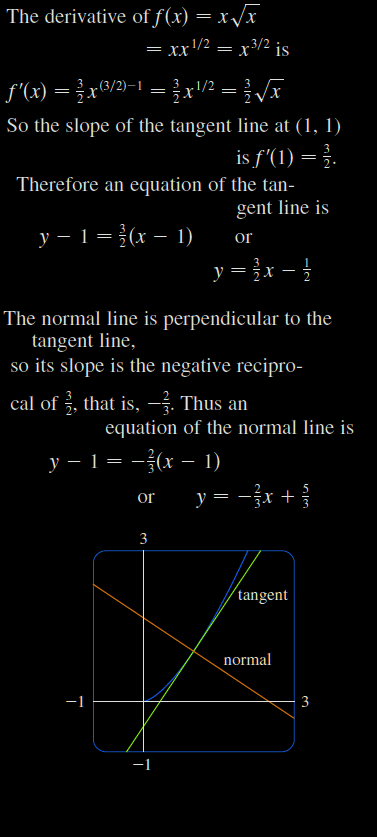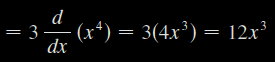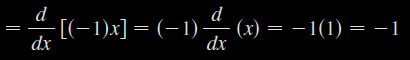# Stewart – Calculus – 3.1 – Derivatives of Polynomials and Exponential Functions

(a)$f(x)=\frac{1}{x^{2}}$

(b)$y=\sqrt[{3}]{x^{2}}$

Find equations of the tangent line and normal line to the curve$y=x\sqrt{x}$ at the point$(1, 1)$. Illustrate by graphing the curve and these lines.

THE CONSTANT MULTIPLE RULE$\frac{d}{\mathit{dx}}(3x^{4})$$\frac{d}{\mathit{dx}}(-x)$